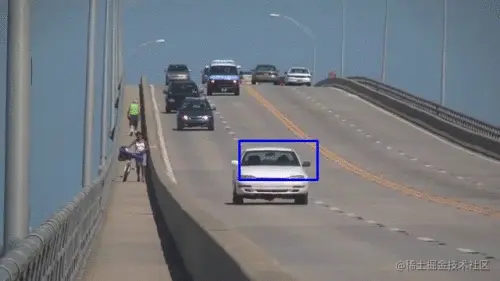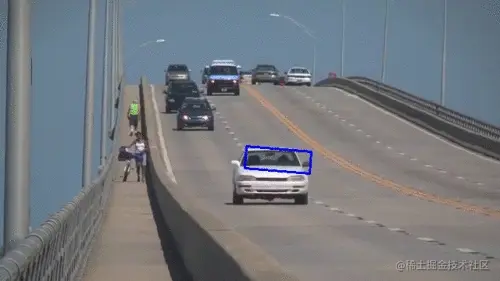# Use python, opencv's meanshift and CAMSHIFT algorithms to find and track objects in video

2022-01-30 09:43:49

# Use Python,OpenCV Of Meanshift and Camshift Algorithm to find and track objects in video

This blog will show you how to use Meanshift and Camshift Algorithm to find and track objects in video .

**MeanShift： Uniform shift

Camshift（Continuously Adaptive Meanshift） Continuous adaptive uniform shift **

• cv2.meanShift(): Meanshift Even shift can always find a window with the largest pixel distribution , And track the object ;

• cv2.CamShift(): CAMshift yes Meanshift The optimization of the , It will continuously automatically resize the window , And calculate the direction of the best fitting ellipse . It again applies the mean transformation with the new scaled search window and the position of the previous window , Until the required accuracy is achieved ;

## 1. design sketch

The official sample ——Meanshift The effect diagram of uniform displacement is as follows ：The official sample ——CAMshift The effect diagram of continuous adaptive average shift is as follows :

You can see Camshift It will automatically adjust the size and rotation of the box , It can better fit the tracked object ;## 2. Source code

### 2.1 MeanShift

``````#  Use MeanShift Average shift sum  CAMshift（Continuously Adaptive Meanshift） Continuously adaptive equalization to find and track objects

# CAMshift  yes  MeanShift The optimization of the , It will continuously automatically resize the window , And calculate the direction of the best fitting ellipse . It again applies the mean transformation with the new scaled search window and the position of the previous window , Until the required accuracy is achieved ;
import numpy as np
import cv2

cap = cv2.VideoCapture('images/slow_traffic_small.mp4')

#  Get the first frame of the video
ret, frame = cap.read()

#  Set the initial window position
x, y, w, h = 300, 200, 100, 50  #  Hard coded location
track_window = (x, y, w, h)

#  Set the tracking object ROI
roi = frame[y:y + h, x:x + w]

#  Only consider HSV The color of
hsv_roi = cv2.cvtColor(roi, cv2.COLOR_BGR2HSV)
#  In order to avoid wrong values due to low light , Use  cv2.inRange()  Function to discard low light value .
mask = cv2.inRange(hsv_roi, np.array((0., 60., 32.)), np.array((180., 255., 255.)))
roi_hist = cv2.calcHist([hsv_roi], , mask, , [0, 180])
cv2.normalize(roi_hist, roi_hist, 0, 255, cv2.NORM_MINMAX)

#  Set termination criteria ,10  Iterations or moves at least  1pt
term_crit = (cv2.TERM_CRITERIA_EPS | cv2.TERM_CRITERIA_COUNT, 10, 1)

while (1):
ret, frame = cap.read()

if ret == True:
hsv = cv2.cvtColor(frame, cv2.COLOR_BGR2HSV)
dst = cv2.calcBackProject([hsv], , roi_hist, [0, 180], 1)

#  application meanshift Get new location
ret, track_window = cv2.meanShift(dst, track_window, term_crit)

#  Draw it on the image
x, y, w, h = track_window
img2 = cv2.rectangle(frame, (x, y), (x + w, y + h), 255, 2)
cv2.imshow('img2', img2)

k = cv2.waitKey(60) & 0xff
if k == 27:
break
else:
cv2.imwrite(chr(k) + ".jpg", img2)

else:
break

cv2.destroyAllWindows()
cap.release()
Copy code ``````

### 2.2 Camshift（Continuously Adaptive Meanshift） Continuous adaptive uniform shift

``````#  Use MeanShift Average shift sum  CAMshift（Continuously Adaptive Meanshift） Continuously adaptive equalization to find and track objects
# CAMshift  yes  MeanShift The optimization of the , It will continuously automatically resize the window , And calculate the direction of the best fitting ellipse . It again applies the mean transformation with the new scaled search window and the position of the previous window , Until the required accuracy is achieved ;

import numpy as np
import cv2

cap = cv2.VideoCapture('images/slow_traffic_small.mp4')

#  Get the first frame of the video
ret, frame = cap.read()

#  Set the initial window position
x, y, w, h = 300, 200, 100, 50  #  Hard coded location
track_window = (x, y, w, h)

#  Set the tracking object ROI
roi = frame[y:y + h, x:x + w]

hsv_roi = cv2.cvtColor(roi, cv2.COLOR_BGR2HSV)
mask = cv2.inRange(hsv_roi, np.array((0., 60., 32.)), np.array((180., 255., 255.)))
roi_hist = cv2.calcHist([hsv_roi], , mask, , [0, 180])
cv2.normalize(roi_hist, roi_hist, 0, 255, cv2.NORM_MINMAX)

#  Set termination conditions , iteration 10 Move once or at least 1pt
term_crit = (cv2.TERM_CRITERIA_EPS | cv2.TERM_CRITERIA_COUNT, 10, 1)

while (1):
ret, frame = cap.read()

if ret == True:
hsv = cv2.cvtColor(frame, cv2.COLOR_BGR2HSV)
dst = cv2.calcBackProject([hsv], , roi_hist, [0, 180], 1)

#  application camshift Get new location
#  Returns a rotated rectangle and box parameter （ Used to pass as a search window in the next iteration ）
#  It first applies the mean transformation . once meanshift convergence , It updates the size of the window , And calculate the direction of the best fitting ellipse . It again applies the mean transformation with the new scaled search window and the position of the previous window . The process continues until the required accuracy is met .
ret, track_window = cv2.CamShift(dst, track_window, term_crit)

#  Draw on the image
pts = cv2.boxPoints(ret)
pts = np.int0(pts)
img2 = cv2.polylines(frame, [pts], True, 255, 2)
cv2.imshow('img2', img2)
# cv2.waitKey(0)

k = cv2.waitKey(60) & 0xff
if k == 27:
break
else:
cv2.imwrite(chr(k) + ".jpg", img2)

else:
break

cv2.destroyAllWindows()
cap.release()
Copy code ``````